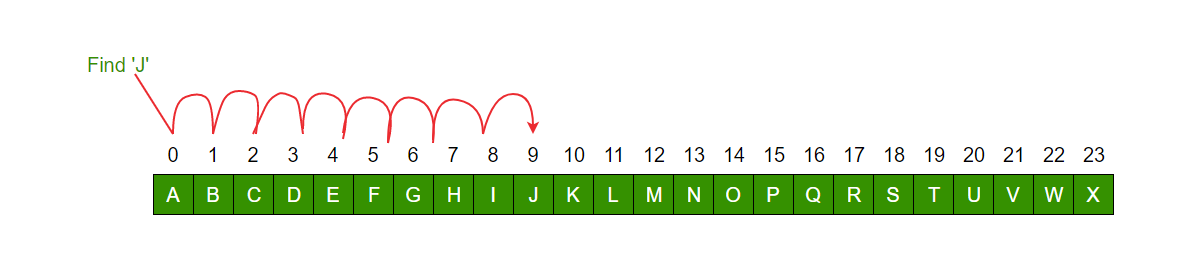# Python Program for Linear Search

• Difficulty Level : Easy
• Last Updated : 22 Jun, 2022

Problem: Given an array arr[] of n elements, write a function to search a given element x in arr[].

Examples :

```Input : arr[] = {10, 20, 80, 30, 60, 50,
110, 100, 130, 170}
x = 110;
Output : 6
Element x is present at index 6

Input : arr[] = {10, 20, 80, 30, 60, 50,
110, 100, 130, 170}
x = 175;
Output : -1
Element x is not present in arr[].```

A simple approach is to do a linear search, i.e

• Start from the leftmost element of arr[] and one by one compare x with each element of arr[]
• If x matches with an element, return the index.
• If x doesn’t match with any of the elements, return -1.

Example:Iterative Approach:

## Python

 `# Searching an element in a list/array in python``# can be simply done using \'in\' operator``# Example:``# if x in arr:``#   print arr.index(x)` `# If you want to implement Linear Search in python` `# Linearly search x in arr[]``# If x is present then return its location``# else return -1` `def` `search(arr, x):` `    ``for` `i ``in` `range``(``len``(arr)):` `        ``if` `arr[i] ``=``=` `x:``            ``return` `i` `    ``return` `-``1`

Recursive Approach:

## Python

 `# This is similar to the above one, with the only difference``# being that it is using the recursive approach instead of iterative.`  `def` `search(arr, curr_index, key):``    ``if` `curr_index ``=``=` `-``1``:``        ``return` `-``1``    ``if` `arr[curr_index] ``=``=` `key:``        ``return` `curr_index``    ``return` `search(arr, curr_index``-``1``, key)`

The time complexity of the above algorithm is O(n).

Auxiliary Space: O(1) for iterative and O(n) for recursive.

Please refer complete article on Linear Search and Difference Between Recursive and Iterative Algorithms for more details!

My Personal Notes arrow_drop_up